Hydraulics Index

Hydraulic system calculations

Introduction

This page includes a small number of basic notes and calculations to enable selection and sizing of various hydraulic components including .

 Pipes Pumps Motors Air Blast Coolers Accumulators Linear Actuators Heat Loss form Reservoir Power loss from pipe friction

The calculations are simplified and should only be used for initial design purposes.    Theoretical ideal values for the pumps and motors are calculated if 1(100%) is included for the efficiency .     Actual efficiency values are obtained from suppliers literature.     Approximate efficiencies are provided in my notes of pumps and motors.

Note effieciency values used on this page are between 0 and 1 to represent efficiencies between 0 and 100%.

I have tried to include supplementary unit equations to identify the factors used, as I have based the units generally on practical working units and not absolute units eg. litres/min as opposed to m3 /s, and bars as opposed to N/m2.    I have also assumed that gauge pressures are used.

I have created a excelcalcs spreadsheet for convenient access to all of the equations found on this page for members of Exelcalcs.com . This is located at ExcelCalcs.com calculation Simple Hydrostatic Transmission system calculations

Units

 l = length (metre) A = pipe dia (metre2 ) di = Pipe inside diameter(mm) V = Volume (metre3 ) q = flow rate (litres /min ) t = time ( s ) ω = angular velocity ( rad /s ) n = rotational speed ( rev/min ) v = velocity ( m /s ) δ = fluid density ( kg / metre3 ) F = Force(N) T = Torque ( N.m ) p = Pressure ( bar = 10^5 Pa = 10^5 N/metre2 ) K = Bulk Modulus ( bar = 10^5 Pa = 10^5 N/metre2 ) μ = Dynamic viscosity (Pa.s ) ν = Kinematic viscosity (metre2.s ) W = Work /Energy (Joule = N.m) Po = Power (W = J/s = Nm/s ) T = Temperature ( deg. C) η = Efficiency

Pipe sizing

The recommended flow velocities for hydraulic fluid are listed below.

ref webpage Pneumatics / Hydraulics

 Pump Suction...0,6 - 1,2 m/s Delivery flow...2,0 - 5,0 m/s Return flow...1,5 - 4,0 m/s

The calculation of the fluid velocity is the flow ( q ) / pipe bore area ( Ai )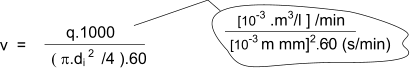q = flow rate (litres/m )
di pipe inside dia (mm)
v = flow velocity (m/s )

Hydraulic Power due to pressure in flowing hydraulic oil

The hydraulic power in the flowing fluid in a hydraulic piping is simply the flow rate (q) x the pressure of the fluid (p)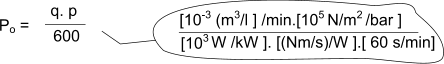q = flow rate (litres/min )
p =pressure (bar)

Hydraulic Pump Calculations

Notes on the various types of positive displacement hydraulic pumps are found on webpage Positive displacement pumps.    The calculations below relate most specifically to Piston pumps, vane pumps and gear pumps operating a pressures in excess of 70 bar.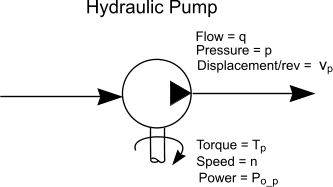The pump fluid delivery is the pump displacement per revolution x the rotational speed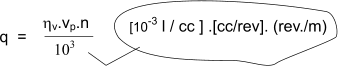>

vp = displacement /rev (cc /rev )
n = revs/min
q = litres /min
ηv = Volumetric Efficiency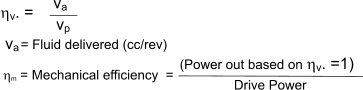The pump drive power required at the shaft =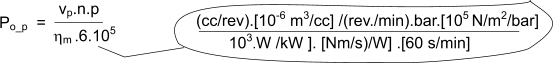vp = displacement /rev (cc /rev )
n = revs/min
p = pressure (bar)
p = pressure (bar)
Shaft power kW
ηm = Mechanical efficiency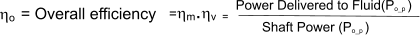Hydraulic Motor Calculations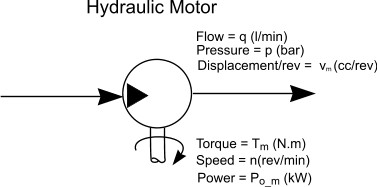The speed is the fluid flow rate q divided the motor displacement/rev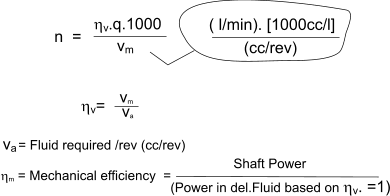vm = displacement /rev (cc /rev )
n = revs/min
q = litres /min
η v = Volumetric efficiency

The motor drive power available at the shaft =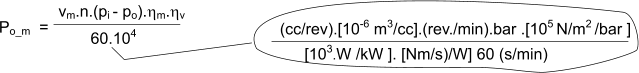vm = displacement /rev (cc /rev )
n = revs/min
pi = input pressure (bar)
po = discharge pressure (bar)
Po=Shaft power kW
η v = Volumetric efficiency
η m = Mechanical efficiency

The motor torque available at the shaft =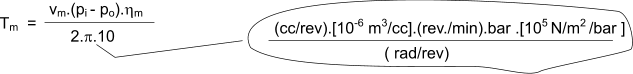vm = displacement /rev (cc /rev )
n = revs/min
pi = input pressure (bar)
po = discharge pressure (bar)
Po=Shaft power kW
η m = Mechanical efficiency

Air Blast Cooler Calculation

Three simple methods of specifying a cooler are provided.

 One simply estimates the cooling requirement based on the efficiency of the hydraulic motor.     This results in the minimum cooler requirement. The second method is based on a cooling requirement as a ratio of the input power .     This is more conservative The third results from testing the system operating conditions to estimate the cooling requirement.

On arriving at a cooling requirement based at a circulating fluid flow rate, the air blast cooler is specified to remove the heat when the oil is at a desired maximum temperature and at the worst case ambient (air) temperature

Heat developed based on motor efficiency

pf = (1- ηm ) . pm / ηm

pf = heat power to fluid
ηm = motor efficiency
pm= motor power output shaft power

Heat developed based on Input power

This simply assumes that the overall efficiency of the system (pumps, motors, and valves result in losses of about 30%)

pf = 0,3 . pe

pf = heat power to fluid
pe= electric motor power /input power

Heat developed based on temperature rise of reservoir

pf = (ΔT. coil. ρoil.V )/ t

pf = heat power to fluid
Δt = Temperature increase (C)
coil = specific heat of oil (kJ/deg.kg)
ρoil = density of oil (kg/m3 )
V = reservoir volume (m3 )
t = time for temperature rise (seconds)

Rating of Air Blast cooler

p01 = m.pf /(T1 - T3)

p01 = Thermal rating of cooler (kW /Degree (C))
m = coiler safety margin
T1 = Desired oil temperature (deg. C)
T3 = Ambient Air Temperature (deg. C)

Hydraulic Accumulator

It may be necessary for an hydraulic system to work with a pump operating at constant speed while the motor or cylinder is not working at a steady speed.     An accumulator acts as an hydraulic flywheel to even out the energy flow and enable a lower pump specification for a given duty.

An accumulator is often placed close to the pump with a non-return valve preventing flow back to the pump, when the accumulator is used to smooth pulsations.       It also helps protect the system from fluid hammer. An additional benefit is the surplus energy that can be stored while the pump is subject to low demand.    The designer can often use a smaller-capacity pump relative to a short term high flow demand.     The outflow of energy from a discharging accumulator can be greater, for a short time, than the pump could develop. The precharge is the pressure of the gas in the accumulator with minimum hydraulic fluid in the fluid side. A gas accumulator is precharged with nitrogen gas, to the desired pressure, when there is no hydraulic fluid in the accumulator.

A rule of thumb for bladder accumulators is to set the precharge pressure to approximately 80% of the desired minimum hydraulic system pressure. A rule of thumb for gas piston accumulators is to set the precharge pressure to approximately of the of the desired minimum hydraulic pressure. hydraulic system pressure.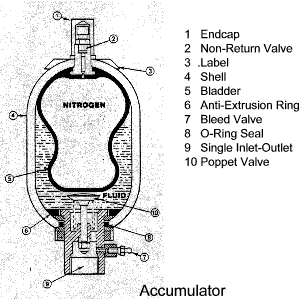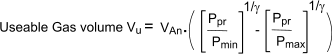The useable volume Vu (litres) is the volume of fluid an accumulator will deliver as the system pressure falls from the maxiumum pressure pmax (bar) to the minimum presssure pmin (bar).

Hydraulic cylinder

The hydraulic force exerted by an extending Hydraulic Cylinder is calculated shown below.    Please not the seal friction and fluid backpressure is not taken into account . The resulting forces will therefore be a little high    The backpressure can easily be accounted for by calculating the resultant extending or retracting force as F1 - F2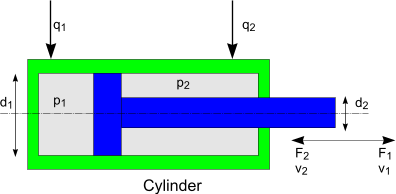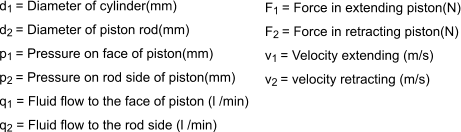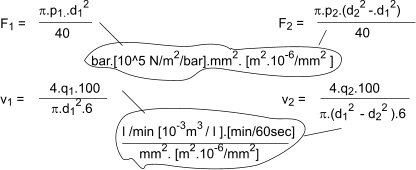The hydraulic force produced by a ram or single acting cylinder is calculated using the same equations as shown below.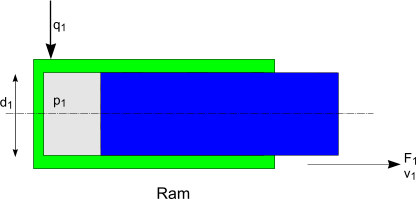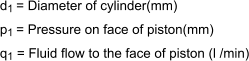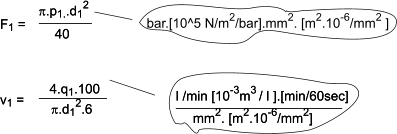Heat Loss From Reservoir

In operating a hydrostatic transmission system comprising pumps and motors and/or actuators there are significant mechanical losses. The result in the hydraulic fluid heating.     If the losses are significant there may be a need to include a cooler in the system to remove the excess heat.     This calculation provides a quick estimate of the natural losses to the environment.    The simple calculation is based on the reservoir being located in an area such that the ambient air can freely circulate over all of its surfaces.    I have obtained the value of C by considering a number of values found on the links below and on my webpage. Thermal design of Gearboxes

Heat loss from reservoir Q = C.(Tf - Ta).A

Q = heat loss (kW)
C = heat loss coefficient. For a steel tank a value of C = 0,0145 kW/ 0C / m2 is reasonable
Tf = Temperature of Fluid (0C)
Tf = Max ambient temperature ( 0C)
A = Area of tank surface exposed to a flow of air (m2)

Approximate Power Loss due to head loss in piping

It is possible to easily calculate the approximate head loss in the pressure piping of a hydrostatic system.     Using the methods identified webpage Pipe Flow

The assumptions made in these calcs are

1) The tubing is smooth bore cds/ cfs

2) The estimate does not include for filters, valves, heights etc.

3) The properties of the oil i.e density and viscosity are approximate

4) The friction values f are approximate.

First it is necessary to calculate the Renold number for the flowing fluid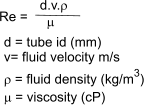Mineral oil as used for hydraulics systems has a density of approx 850 kg/m3
I list a few approx. viscosity values below

 oil grade Viscosity cP at 40o C at 100 o C Hydraulic oil ISO 32 27 4.7 Hydraulic oil ISO 46 39 5.9 Hydraulic oil ISO68 57 8.7 Hydraulic oil ISO 100 82 9.4 Hydraulic oil ISO 150 125 12

It is normal to use a Moony chart to evaluate the friction factor f based on the tube roughness and the Renold number.

To simplify this process I have determined some approximate friction factors based on a range of Re numbers and assuming the tube is smooth bored

 Reynolds Number range Friction factor (f) 0-2500 64/Re 2500 - 104 0,03 104 -105 0,02 105 -106 0,016

The head loss if fluid metres is simply calculated as follows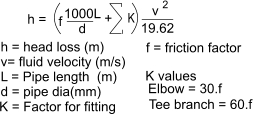It will be necessary to detemine the flow path from the pump to the actuator estimate the pipe length and number of elbows and tees to complete the calculation for the pressure side. Then repeat the process for the return route which should have lower velocities and a larger diameter

The pressure loss and the power loss is calculated from the total pipe head loss as follows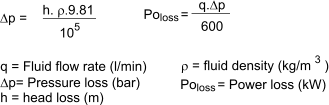Example :
Consider a hydrostatic power transmission system. The tube from the pump to the motor is 20mm i.dia, 20m long, and includes 3 elbows and 2 tees .     The flow rate is 50 litres/min and the oil is grade ISO 68 at 40 deg.C

Viscosity = 57cP, Density = 850 kg/m3.

Fluid velocity = 2,65m/s Using equation at Pipes

Re Number = d.v.ρ /μ=795 (laminer) and as a result f = 0,081

head loss h = ( f.1000.L/d + 3.f.30 + 2 .f* 60).v2 /19.62 = 35m

Pressure loss ΔP= h.ρ.9.81/ 10^5 = 2,91 bar

Power loss = Q.ΔP/600 = 0,24 kW

The return leg from the motor to the reservoir is 25 mm i.dia, 20m long, and includes 3 elbows and 2 tees .     The flow rate is 50 litres/min and the oil is grade ISO 68 at 40 deg.C

Viscosity = 57cP, Density = 850 kg/m3.

Fluid velocity = 1,7 m/s Using equation at Pipes

Re Number = d.v.ρ /μ=632 (laminer) and as a result f = 0,101

head loss h = ( f.1000.L/d + 3.f.30 + 2 .f* 60).v2 /19.62 = 15m

Pressure loss ΔP= h.ρ.9.81/ 10^5 = 1,25 bar

Power loss = Q.ΔP/600 = 0,10 kW

Total power loss as pipe resistance = 0,24 + 0,10 = 0.34 kW.

 Useful Links E4 Training- Hydraulic Calculators.. A number of useful calculators and notes. Hydra Products. Calculators.. Equipment supplier .A number of useful calculators and notes. Parker .. Supplier of wide range of hydraulic and pneumatic components. And a great deal of useful information Hydraulics Technical Library.. Online hydraulics library containing application formulas, product data, maintenance tips and "how-to" tutorials. How stuff works Hydraulics.. Clear useful basic information.. Eaton .. A supplier of hydraulic equipment.. Lift Systems...High capacity lifting using hydraulics Hydraulics...A detailed document on all aspects of Hydraulics IHS service -Pipe sizes...Downloadable paper titled Determining Tube Size for Hydraulic Systems.
Hydraulics Index